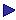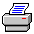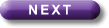Tutorial Contents Tutorial Six: Predicate Calculus with Identity - TRussell's Theory of Descriptions - Scope - Tableau Rules for Identity More Tutorials OneTwoThreeFourFiveSeven# 6: Predicate Calculus with Identity

 Identity and numbers of things

We know how to say there is at least one cow: \$x x is a cow. Or, if we use "Cx" for "x is a cow": \$xCx.

"\$x\$y[CxÙCy]" still means "There is at least one cow".

To say that there are at least two cows we need to make use of the notion of identity. We use "x=y" to mean that x is one and the same thing as y. (So "The Prime Minister = Tony Blair" paraphrases "The Prime Minister is Tony Blair".

"x=y" is a 2-place predicate. It behaves just like other 2-place predicates in formulae, the only difference being that the predicate letter goes in the middle instead of at the beginning.

Armed with this we can formalise "There are at least two cows" thus:

\$x\$y[[CxÙCy]Ù¬x=y]

Notice that we do not surround "x=y" with brackets, and that the "¬" goes with "x=y"; it does NOT negate "x"  and an expression such as "x= ¬y" is NONSENSE.

The trick was to say, so to speak, that the x and the y were different things: so there had to be at least two cows. So to say that there are at least three cows we simply use "x", "y" and "z" (say) and say that the x and the y are different things, the x and the z are different things, and the y and the z are different. Thus:

\$x\$y\$z[[CxÙ[CyÙCz]]Ù [¬x=yÙ[¬x=zÙ¬y=z]]]

To say that there is at most one cow we can simply negate the formula which said that there were at least two (if there are not at least two, there must be at most one):

¬\$x\$y[[CxÙCy]Ù¬x=y]

Another way of saying the same thing is:

"x"y[[CxÙCy]®x=y]

(This says that if x is a cow and y is a cow, then x is one and the same as y: that is, there is at most one cow.)

To see that formulae do indeed say the same thing, remember the idea that if you push a "¬" through a quantifier, it flips to the other sort of quantifier. So doing this to "¬\$x\$y[[CxÙCy]Ù¬x=y]", we get:

"x"y¬[[CxÙCy]Ù¬x=y].

Then we remember that "¬[jÙ¬y]" is equivalent to "[j®y]". Applying this we get:

"x"y[[CxÙCy]®x=y].

How do we say that there is exactly one cow? One way would be to say that there is at least one cow and there is at most one cow:

[\$xCxÙ¬\$x\$y[[CxÙCy]Ù¬x=y]].

More compactly we could say:

\$x[CxÙ¬\$y[CyÙ¬x=y]].

(i.e, there is a least one cow, and there isn't another one.) Or:

\$x[CxÙ"y[Cy®x=y]].

Even more compactly one could say:

\$x"y[x=y«Cy].

But you are strongly advised not to, because it is not very obvious that this does mean the right thing; and, as a result, it is rather easy to get it slightly wrong and write:

*\$x"y[x=y«Cx]

which means something quite different: it means that there is just one thing in the universe, and it is a cow.

To see that "\$x"y[x=y«Cy]" does indeed mean that there is exactly one cow, notice that it is equivalent to:

\$x"y[[x=y®Cy]Ù[Cy®x=y]],

where the first bit is a funny way of saying that there is a cow ("there is an x such that anything which is the same thing as it is a cow"), and the second bit says that there isn't another one.

"\$x"y[x=y«Cx]", on the other hand, is equivalent to:

\$x"y[[x=y®Cx]Ù[Cx®x=y]],

where the first bit is another funny way of saying that there is a cow ("there is an x such that if anything is one and the same thing as it, x is a cow"), and the second bit says that there is something such that if it is a cow then everything is one and the same thing as it, which means that there is just one thing altogether, because there is something which is a cow (according to the first bit), and so everything must be the same thing as it: i.e. there is just one thing  the cow.

Once we can say that there is exactly one cow, we can formalise other things that say that there is exactly one something. So we can paraphrase

Farmer Giles owns exactly one brown cow

as

There is exactly one brown-cow-that-Farmer-Giles-owns.

So:

\$x[[CxÙ[BxÙOax]]Ù"y[[CyÙ[ByÙOay]]®y=x]]

Where we are using this interpretation:

Domain: everything

Cx: x is a cow

Bx: x is brown

Oxy: x owns y

a: Farmer GilesPrint this page